# Bivector

(diff) ← Older revision | Latest revision (diff) | Newer revision → (diff)

A class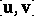of ordered pairs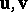of vectors of an affine space, starting at a common origin (considered in a basis of the underlying space). A bivector is considered to be equal to zero if its constituent vectorsand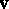are collinear. A non-zero bivector generates a unique two-dimensional space in, its carrier. Two bivectors are said to be parallel if their carrier planes are parallel. Ifhas finite dimension, andare the contravariant coordinates of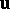, while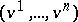are the contravariant coordinates of, calculated with respect to some basisof the underlying space of, then the quantities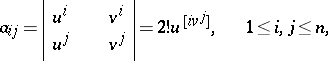are called the Plücker coordinates of the pair. Two pairs of vectors are in the same class if their Plücker coordinates with respect to some basis coincide (they will then be equal in any basis). The coordinates of the class are then called the coordinates of the bivectorwith respect to the basis. These coordinates are skew-symmetric with respect to their indices; they contain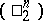independent coordinates. Under a transition to another basis of, the coordinates of a bivector behave as coordinates of a twice-contravariant tensor. A bivector is also called a free bivector. In the presence of a scalar product in, a number of metrical concepts of vector algebra can be extended to bivectors. The measure of a bivector is the area of the parallelogram formed by the vectors, the origin of each one being located in the end of the preceding one. This only depends on the class, not on the representatives. The scalar product of two bivectors is the number equal to the product of the measures of the factors by the cosine of the angle between their two carrier planes. This product is a bilinear form of the coordinates of the factors, the coefficients of which are defined by the metric tensor of the spacealone.

If the dimension ofis 3, the bivectormay be identified with a vector ofwhich, in the presence of a scalar product, is called the vector product of the vectors.

In tensor calculus a bivector is an arbitrary contravariant skew-symmetric tensor of valency 2 (i.e. a tensor of type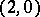). Each such tensor may be represented as a sum of tensors, to which correspond non-zero bivectors in the above sense with different carrier planes. They define the sheets of the bivector. The rank of the skew-symmetric matrix of dimension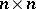consisting of the coordinates of a bivector is an even number, whereis the number of sheets of the bivector. In a real affine spacethis matrix is similar to the matrixwith the blocks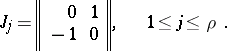How to Cite This Entry:
Bivector. Encyclopedia of Mathematics. URL: http://encyclopediaofmath.org/index.php?title=Bivector&oldid=18904
This article was adapted from an original article by L.P. Kuptsov (originator), which appeared in Encyclopedia of Mathematics - ISBN 1402006098. See original article